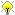# Some relative number fields arithmetic benchmarks

These examples have the patch from trac 9500 applied.

## An example where no reduction is needed -- we just multiply the generators

```sage: R.<x> = QQ[]
sage: K.<a,b> = NumberField([x^19 + 17*x^3 + 2*x - 3, x^3 + 2*x - 17])
sage: R.<a0,b0> = QQ[]
sage: S.<aa,bb> = R.quotient([a0^5 + 17*a0^3 + 2*a0 - 3, b0^3 + 2*b0 - 17])
sage: timeit('a*b')
125 loops, best of 3: 3.79 ms per loop
sage: timeit('aa * bb')
625 loops, best of 3: 45.2 µs per loop
sage: 3.79 / 0.0452
83.8495575221239
sage: time c = a/b
Time: CPU 29.65 s, Wall: 30.35 s
sage: time cc = aa / bb
Time: CPU 0.00 s, Wall: 0.00 s
sage: timeit('aa/bb')
625 loops, best of 3: 262 µs per loop
sage: 29.65/0.000262
113167.938931298
sage: time z = [aa/bb for i in [1..1000]]
Time: CPU 0.27 s, Wall: 0.28 s
sage: time z = [aa*bb for i in [1..1000]]
Time: CPU 0.05 s, Wall: 0.05 s
sage: gb = S.defining_ideal().groebner_basis()
sage: time z = [(a0*b0).reduce(gb) for i in [1..100000]]
Time: CPU 0.89 s, Wall: 1.19 s```

Scary -- so quotient polynomial rings give a speedup by a factor of over 100000for division in this example. This is worth doing.

Compare to Magma:

```R<x> := PolynomialRing(RationalField());
K<a,b>:= NumberField([x^19 + 17*x^3 + 2*x - 3, x^3 + 2*x - 17]);
time z := [a*b : i in [1..100000]];
Time: 0.750
time z := [a/b : i in [1..1000]];
Time: 0.140```

In this example, Sage is a little slower, but not by much.

## An example with a healthy sized element, so multiplication requires reduction

Sage right now is very slow:

```sage: R.<x> = QQ[]
sage: K.<a,b> = NumberField([x^19 + 17*x^3 + 2*x - 3, x^3 + 2*x - 17])
sage: z = (a+b)^20
sage: timeit('z*z')
125 loops, best of 3: 3.27 ms per loop
sage: time n = 1/z
Time: CPU 28.69 s, Wall: 29.23 s```

We get a massive speedup (over 100 times):

```sage: R.<a0,b0> = QQ[]
sage: S.<aa,bb> = R.quotient([a0^5 + 17*a0^3 + 2*a0 - 3, b0^3 + 2*b0 - 17])
sage: zz = (aa+bb)^20
sage: ww = zz.lift()
sage: timeit('zz*zz')
625 loops, best of 3: 275 µs per loop
sage: timeit('1/zz')
5 loops, best of 3: 247 ms per loop
sage: time v = [zz*zz for i in [1..1000]]
Time: CPU 0.30 s, Wall: 0.30 s
sage: time v = [1/zz for i in [1..10]]
Time: CPU 2.47 s, Wall: 2.49 s
sage: 28.69 / .247
116.153846153846```

Sage is better than Magma at multiplication, but over 10 times slower and division (by default):

```R<x> := PolynomialRing(RationalField());
K<a,b>:= NumberField([x^19 + 17*x^3 + 2*x - 3, x^3 + 2*x - 17]);
z := (a+b)^20;
time v := [z*z : i in [1..1000]];
Time: 0.440
time v := [1/z : i in [1..10]];
Time: 0.190
>>> 2.47/.19
13.0000000000000```

days23.5/projects/relative_number_fields (last edited 2010-07-14 19:37:22 by was)